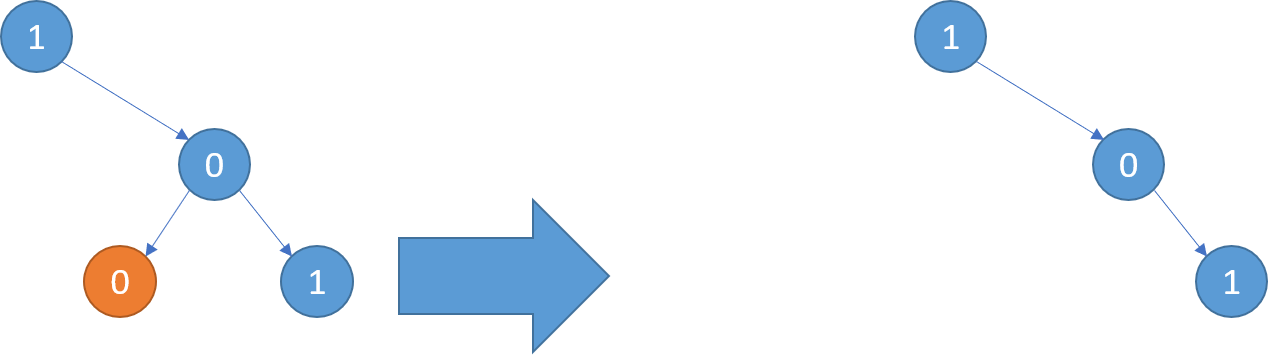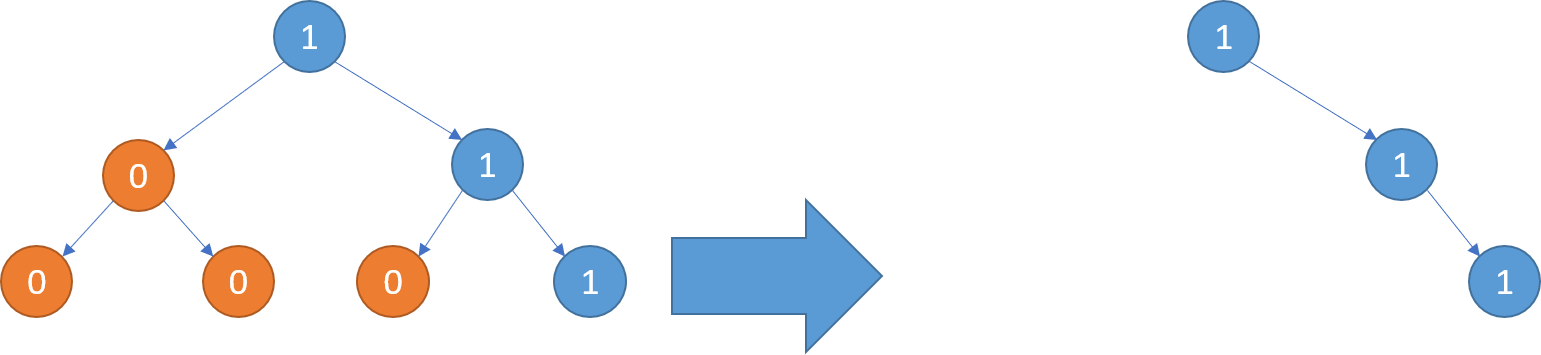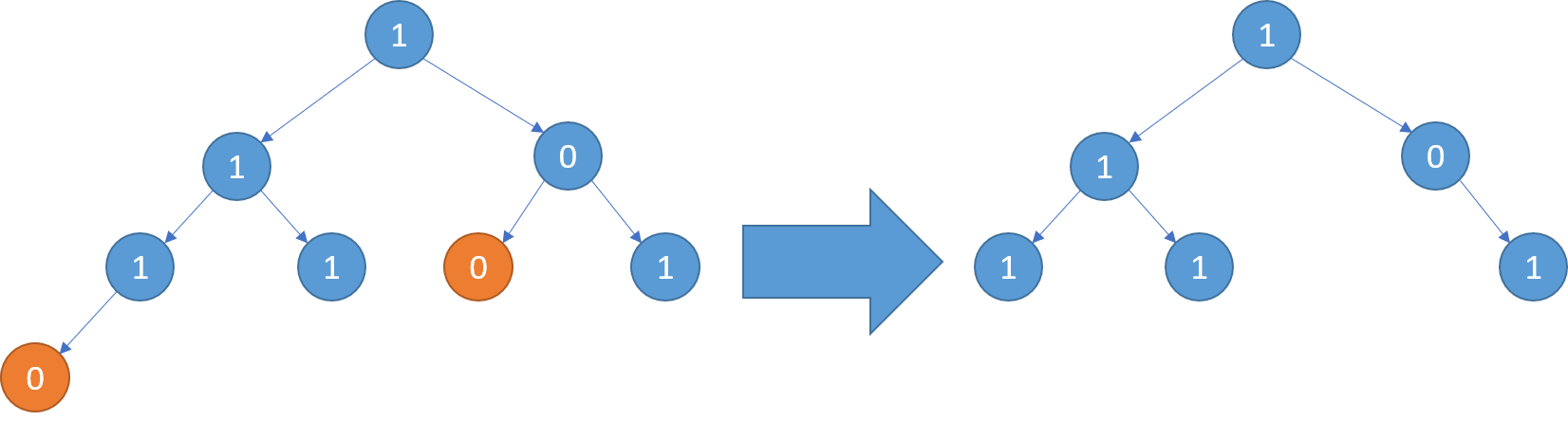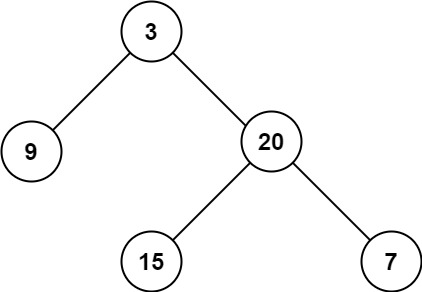# Posts published in “Recursion”

Serialization is the process of converting a data structure or object into a sequence of bits so that it can be stored in a file or memory buffer, or transmitted across a network connection link to be reconstructed later in the same or another computer environment.

Design an algorithm to serialize and deserialize a binary tree. There is no restriction on how your serialization/deserialization algorithm should work. You just need to ensure that a binary tree can be serialized to a string and this string can be deserialized to the original tree structure.

Clarification: The input/output format is the same as how LeetCode serializes a binary tree. You do not necessarily need to follow this format, so please be creative and come up with different approaches yourself.

Example 1:

```Input: root = [1,2,3,null,null,4,5]
Output: [1,2,3,null,null,4,5]
```

Example 2:

```Input: root = []
Output: []
```

Example 3:

```Input: root = 
Output: 
```

Example 4:

```Input: root = [1,2]
Output: [1,2]
```

Constraints:

• The number of nodes in the tree is in the range `[0, 104]`.
• `-1000 <= Node.val <= 1000`

Idea: (Recursion)

```We can encodes the tree to a single string.
// # indicates reach to the leave
Convert above tree to 12##34##56## ```

Time Complexity O(n)

Solution:

``````/**
* Definition for a binary tree node.
* function TreeNode(val) {
*     this.val = val;
*     this.left = this.right = null;
* }
*/

/**
* Encodes a tree to a single string.
*
* @param {TreeNode} root
* @return {string}
*/
var serialize = function(root) {
let res = [];
serializer(root, res);
return res.join(",");
};

var serializer = function(root, output) {
if (root === null) {
output.push("#");
return;
}
output.push(root.val);
serializer(root.left, output);
serializer(root.right, output);
}

// Decodes your encoded data to tree.
var deserialize = function(data) {
data = data.split(",");
let index = 0;

function deserializer(data) {
if(index > data.length || data[index] === "#") {
return null;
}
let root = new TreeNode(parseInt(data[index]));
index++;
root.left = deserializer(data);
index++;
root.right = deserializer(data);
return root;
}
return deserializer(data);
};``````

Given a string containing digits from `2-9` inclusive, return all possible letter combinations that the number could represent. Return the answer in any order.

A mapping of digit to letters (just like on the telephone buttons) is given below. Note that 1 does not map to any letters.

Example 1:

```Input: digits = "23"
```

Example 2:

```Input: digits = ""
Output: []
```

Example 3:

```Input: digits = "2"
Output: ["a","b","c"]
```

Constraints:

• `0 <= digits.length <= 4`
• `digits[i]` is a digit in the range `['2', '9']`.

Idea:

Use DFS template

Solution:

``````/**
* @param {string} digits
* @return {string[]}
*/
var letterCombinations = function(digits) {
let res = [];
if (digits.length === 0) return res;
// you can use array or use hashmap
const nums = [];
nums = ['a','b','c'];
nums = ['d','e','f'];
nums = ['g','h','i'];
nums = ['j','k','l'];
nums = ['m','n','o'];
nums = ['p','q','r','s'];
nums = ['t','u','v'];
nums = ['w','x','y','z'];
dfs(res, 0, "", nums, digits);
return res;
};

function dfs(res, start, cur, nums, digits) {
// exit recursive conditon
if (cur.length === digits.length) {
res.push(cur);
return;
}
// possible solution
let possibleLetters = nums[digits[start]];
for (let letter of possibleLetters) {
// modify: add the letter to our current solution
cur += letter;
dfs(res, start + 1, cur, nums, digits);
// recover: backtrack by removing the letter
// before moving onto the next
cur = cur.substring(0, cur.length - 1);
}
}``````

You are given the `root` of a binary search tree (BST) and an integer `val`.

Find the node in the BST that the node’s value equals `val` and return the subtree rooted with that node. If such a node does not exist, return `null`.

Example 1:

```Input: root = [4,2,7,1,3], val = 2
Output: [2,1,3]
```

Example 2:

```Input: root = [4,2,7,1,3], val = 5
Output: []
```

Constraints:

• The number of nodes in the tree is in the range `[1, 5000]`.
• `1 <= Node.val <= 107`
• `root` is a binary search tree.
• `1 <= val <= 107`

Solution:

``````/**
* Definition for a binary tree node.
* function TreeNode(val, left, right) {
*     this.val = (val===undefined ? 0 : val)
*     this.left = (left===undefined ? null : left)
*     this.right = (right===undefined ? null : right)
* }
*/
/**
* @param {TreeNode} root
* @param {number} val
* @return {TreeNode}
*/
var searchBST = function(root, val) {
if (root === null) return null
if (root.val === val)
return root;
else if (val < root.val)
return searchBST(root.left, val);
else
return searchBST(root.right, val);
};``````

We are given the head node `root` of a binary tree, where additionally every node’s value is either a 0 or a 1.

Return the same tree where every subtree (of the given tree) not containing a 1 has been removed.

(Recall that the subtree of a node X is X, plus every node that is a descendant of X.)

```Example 1:
Input: [1,null,0,0,1]
Output: [1,null,0,null,1]

Explanation:
Only the red nodes satisfy the property "every subtree not containing a 1".
The diagram on the right represents the answer.```
```Example 2:
Input: [1,0,1,0,0,0,1]
Output: [1,null,1,null,1]```
```Example 3:
Input: [1,1,0,1,1,0,1,0]
Output: [1,1,0,1,1,null,1]```

Note:

• The binary tree will have at most `200 nodes`.
• The value of each node will only be `0` or `1`.

Solution: (Recursion)

``````/**
* Definition for a binary tree node.
* function TreeNode(val, left, right) {
*     this.val = (val===undefined ? 0 : val)
*     this.left = (left===undefined ? null : left)
*     this.right = (right===undefined ? null : right)
* }
*/
/**
* @param {TreeNode} root
* @return {TreeNode}
*/
var pruneTree = function(root) {
// basic case:
if (root === null) return root;
root.left = pruneTree(root.left);
root.right = pruneTree(root.right);
// found 1 or has children then no need to remove
if (root.val === 1 || root.left || root.right)
return root;
else // remove node
return null;
};``````

Given a binary tree `root` and an integer `target`, delete all the leaf nodes with value `target`.

Note that once you delete a leaf node with value `target`if it’s parent node becomes a leaf node and has the value `target`, it should also be deleted (you need to continue doing that until you can’t).

Example 1:

```Input: root = [1,2,3,2,null,2,4], target = 2
Output: [1,null,3,null,4]
Explanation: Leaf nodes in green with value (target = 2) are removed (Picture in left).
After removing, new nodes become leaf nodes with value (target = 2) (Picture in center).
```

Example 2:

```Input: root = [1,3,3,3,2], target = 3
Output: [1,3,null,null,2]
```

Example 3:

```Input: root = [1,2,null,2,null,2], target = 2
Output: 
Explanation: Leaf nodes in green with value (target = 2) are removed at each step.
```

Example 4:

```Input: root = [1,1,1], target = 1
Output: []
```

Example 5:

```Input: root = [1,2,3], target = 1
Output: [1,2,3]
```

Constraints:

• `1 <= target <= 1000`
• The given binary tree will have between `1` and `3000` nodes.
• Each node’s value is between `[1, 1000]`.

Solution:

``````/**
* Definition for a binary tree node.
* function TreeNode(val, left, right) {
*     this.val = (val===undefined ? 0 : val)
*     this.left = (left===undefined ? null : left)
*     this.right = (right===undefined ? null : right)
* }
*/
/**
* @param {TreeNode} root
* @param {number} target
* @return {TreeNode}
*/
var removeLeafNodes = function(root, target) {
if (root === null) return null;
root.left = removeLeafNodes(root.left, target);
root.right = removeLeafNodes(root.right, target);
// return null to remove that leaf
if (root.val === target && root.left === null && root.right === null) {
return null;
}
return root;
};``````

Given a binary tree, find the lowest common ancestor (LCA) of two given nodes in the tree.

According to the definition of LCA on Wikipedia: “The lowest common ancestor is defined between two nodes `p` and `q` as the lowest node in `T` that has both `p` and `q` as descendants (where we allow a node to be a descendant of itself).”

Example 1:

```Input: root = [3,5,1,6,2,0,8,null,null,7,4], p = 5, q = 1
Output: 3
Explanation: The LCA of nodes 5 and 1 is 3.
```

Example 2:

```Input: root = [3,5,1,6,2,0,8,null,null,7,4], p = 5, q = 4
Output: 5
Explanation: The LCA of nodes 5 and 4 is 5, since a node can be a descendant of itself according to the LCA definition.
```

Example 3:

```Input: root = [1,2], p = 1, q = 2
Output: 1
```

Constraints:

• The number of nodes in the tree is in the range `[2, 105]`.
• `-109 <= Node.val <= 109`
• All `Node.val` are unique.
• `p != q`
• `p` and `q` will exist in the tree.

Solution:

``````/**
* Definition for a binary tree node.
* function TreeNode(val) {
*     this.val = val;
*     this.left = this.right = null;
* }
*/
/**
* @param {TreeNode} root
* @param {TreeNode} p
* @param {TreeNode} q
* @return {TreeNode}
*/
var lowestCommonAncestor = function(root, p, q) {
if (root === null || root === p || root === q) return root;
let left = lowestCommonAncestor(root.left, p, q);
let right = lowestCommonAncestor(root.right, p, q);
return left === null ? right : (right === null ? left : root);
};``````

Given the `root` of a binary tree, invert the tree, and return its root.

Example 1:

```Input: root = [4,2,7,1,3,6,9]
Output: [4,7,2,9,6,3,1]
```

Example 2:

```Input: root = [2,1,3]
Output: [2,3,1]
```

Example 3:

```Input: root = []
Output: []
```

Constraints:

• The number of nodes in the tree is in the range `[0, 100]`.
• `-100 <= Node.val <= 100`

Solution:

``````/**
* Definition for a binary tree node.
* function TreeNode(val, left, right) {
*     this.val = (val===undefined ? 0 : val)
*     this.left = (left===undefined ? null : left)
*     this.right = (right===undefined ? null : right)
* }
*/
/**
* @param {TreeNode} root
* @return {TreeNode}
*/
var invertTree = function(root) {
if (root === null) return root;

let temp = new TreeNode();
temp = root.right;
root.right = root.left;
root.left = tmp;

root.left = invertTree(root.left);
root.right = invertTree(root.right);
return root;
};``````

Given the `root` of a binary tree, return its maximum depth.

A binary tree’s maximum depth is the number of nodes along the longest path from the root node down to the farthest leaf node.

Example 1:```Input: root = [3,9,20,null,null,15,7]
Output: 3
```

Example 2:

```Input: root = [1,null,2]
Output: 2
```

Example 3:

```Input: root = []
Output: 0
```

Example 4:

```Input: root = 
Output: 1
```

Constraints:

• The number of nodes in the tree is in the range `[0, 104]`.
• `-100 <= Node.val <= 100`

Solution:

``````/**
* Definition for a binary tree node.
* function TreeNode(val, left, right) {
*     this.val = (val===undefined ? 0 : val)
*     this.left = (left===undefined ? null : left)
*     this.right = (right===undefined ? null : right)
* }
*/
/**
* @param {TreeNode} root
* @return {number}
*/
var maxDepth = function(root) {
if (root === null) return 0;
return 1 + Math.max(maxDepth(root.left) , maxDepth(root.right));
};``````

Given the `root` of a binary tree, check whether it is a mirror of itself (i.e., symmetric around its center).

Example 1:

```Input: root = [1,2,2,3,4,4,3]
Output: true
```

Example 2:

```Input: root = [1,2,2,null,3,null,3]
Output: false
```

Constraints:

• The number of nodes in the tree is in the range `[1, 1000]`.
• `-100 <= Node.val <= 100`

Solution:

``````/**
* Definition for a binary tree node.
* function TreeNode(val, left, right) {
*     this.val = (val===undefined ? 0 : val)
*     this.left = (left===undefined ? null : left)
*     this.right = (right===undefined ? null : right)
* }
*/
/**
* @param {TreeNode} root
* @return {boolean}
*/
var isSymmetric = function(root) {
return isSameTree(root, root);
};

function isSameTree(t1, t2) {
if (t1 === null && t2 === null) return true;
if (t1 === null || t2 === null) return false;
return t1.val === t2.val && isSameTree(t1.left, t2.right) && isSameTree(t1.right, t2.left);
}``````

Merge two sorted linked lists and return it as a sorted list. The list should be made by splicing together the nodes of the first two lists.

Example 1:

```Input: l1 = [1,2,4], l2 = [1,3,4]
Output: [1,1,2,3,4,4]
```

Example 2:

```Input: l1 = [], l2 = []
Output: []
```

Example 3:

```Input: l1 = [], l2 = 
Output: 
```

Constraints:

• The number of nodes in both lists is in the range `[0, 50]`.
• `-100 <= Node.val <= 100`
• Both `l1` and `l2` are sorted in non-decreasing order.

Solution: (Recursion)

``````/**
* function ListNode(val, next) {
*     this.val = (val===undefined ? 0 : val)
*     this.next = (next===undefined ? null : next)
* }
*/
/**
* @param {ListNode} l1
* @param {ListNode} l2
* @return {ListNode}
*/
var mergeTwoLists = function(l1, l2) {
// If one of the list is emptry, return the other one.
if (!l1 || !l2) return l1 ? l1 : l2;

// The smaller one becomes the head.
if (l1.val < l2.val) {
l1.next = mergeTwoLists(l1.next, l2);
return l1;
} else {
l2.next = mergeTwoLists(l1, l2.next);
return l2;
}
};``````